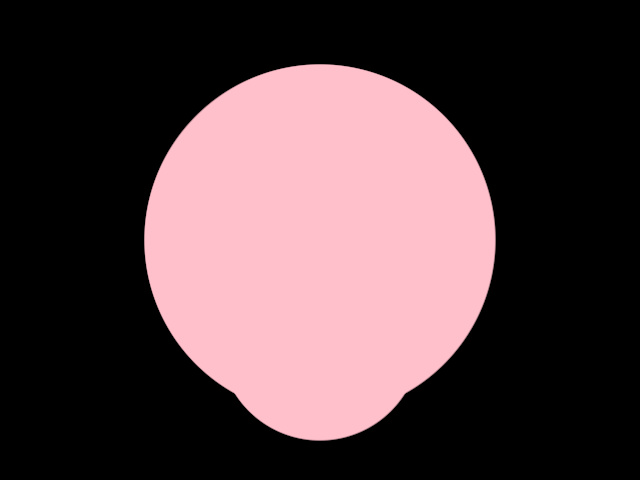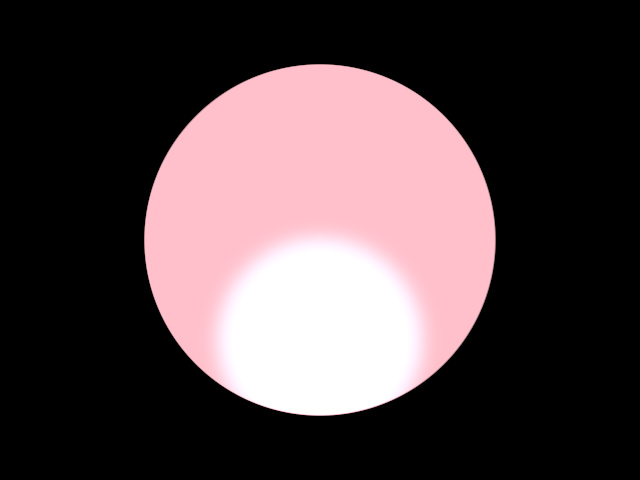# Compositing using orx-compositor

orx-compositor offers a simple DSL for the creation of layered graphics. The compositor manages blending and post-processing of layers for you.

`orx-compositor` works well together with `orx-fx`, `orx-gui`, and `orx-olive`, although they are not a required combination it is worth checking out what the combination has to offer.

## Prerequisites

Assuming you are working on an `openrndr-template` based project, all you have to do is enable `orx-compositor` in the `orxFeatures` set in `build.gradle.kts` and reimport the gradle project.

## Workflow

Let us now work through the workflow of `orx-compositor`. One usually starts with an OPENRNDR skeleton program:

``````fun main() = application {
program {
extend {
}
}
}
``````

Which by itself, of course, does nothing. Let’s extend this skeleton a bit and add the basics for layered graphics. We add a `composite` using `compose {}` and we make sure that our OPENRNDR program draws it on refresh.

Note, if this fails you can fix it by adding `import org.openrndr.extra.compositor.draw`.

``````fun main() = application {
program {
val composite = compose {
}

extend {
composite.draw(drawer)
}
}
}
``````

Now let’s draw something. We do this by adding a `draw {}` inside the `compose {}`. Here we see we use `drawer` like we would use it normally (it is captured in the closure). We also added a `println` to demonstrate that the code inside `compose {}` is executed once, however, code inside `draw {}` is executed every time the composite is drawn.

``````fun main() = application {
program {
val composite = compose {
println("this is only executed once")

draw {
drawer.fill = ColorRGBa.PINK
drawer.stroke = null
drawer.circle(width / 2.0, height / 2.0, 175.0)
}
}

extend {
composite.draw(drawer)
}
}
}
``````

Let’s get to what `orx-compositor` promises: layered graphics. We do this by adding a `layer {}` inside our composite, and inside this layer we add another `draw`.

Every layer has an isolated draw state to prevent users from leaking draw state.

``````fun main() = application {
program {
val composite = compose {
draw {
drawer.fill = ColorRGBa.PINK
drawer.stroke = null
drawer.circle(width / 2.0, height / 2.0, 175.00)
}

layer {
draw {
drawer.fill = ColorRGBa.PINK
drawer.stroke = null
drawer.circle(width / 2.0, height / 2.0 + 100.0, 100.0)
}
}
}

extend {
composite.draw(drawer)
}
}
}
``````

This produces:You may be thinking: “yeah great, we added all that extra structure to the code, but it doesn’t do a single thing that could not be achieved by drawing two circles consecutively”. And you’re right. However, there are now two things we can add with ease: blends and posts. Here a blend describes how a layer’s contents should be combined with the layer it covers, and a post a filter that is applied after the contents have been drawn.

Let’s add a blend and a post to our layer and see what it does:

``````fun main() = application {
program {
val composite = compose {
draw {
drawer.fill = ColorRGBa.PINK
drawer.stroke = null
drawer.circle(width / 2.0, height / 2.0, 175.0)
}

layer {
clip = true
}
draw {
drawer.fill = ColorRGBa.PINK
drawer.stroke = null
drawer.circle(width / 2.0, height / 2.0 + 100.0, 100.0)
}
post(ApproximateGaussianBlur()) {
window = 25
sigma = 10.00
}
}
}
extend {
composite.draw(drawer)
}
}
}
``````

The output:We now see a couple of differences. The smaller circle is blurred while the larger circle is not; The area where the two circles overlap is brighter; The smaller circle is clipped against the larger circle.

These are a results that are not as easily replicated without `orx-compositor`.

Note that the parameters for the post filters (and blend) can be animated, just as the layers contents can be animated:

``````fun main() = application {
program {
val composite = compose {
draw {
drawer.fill = ColorRGBa.PINK
drawer.stroke = null
drawer.circle(width / 2.0 + sin(seconds * 2) * 100.0, height / 2.0, 175.0)
}

layer {
clip = true
}
draw {
drawer.fill = ColorRGBa.PINK
drawer.stroke = null
drawer.circle(width / 2.0, height / 2.0 + cos(seconds * 2) * 100.0, 100.0)
}
post(ApproximateGaussianBlur()) {
// -- this is actually a function that is called for every draw
window = 25
sigma = cos(seconds) * 10.0 + 10.01
}
}
}
extend {
composite.draw(drawer)
}
}
}
``````

## Common use-cases

In this case we have a text and an image that we only want to draw where there is text. This can be achieved by using nested layers and a `Normal` blend with `clip` enabled.

``````fun main() = application {
program {
val composite = compose {
draw {
drawer.clear(ColorRGBa.PINK)
}
layer {
// -- we nest layers to prevent the text layer to be blend with the background
// -- before it is blend with the image layer
layer {
// -- notice how we load the font inside the layer
// -- this only happens once
draw {
drawer.fill = ColorRGBa.WHITE
drawer.fontMap = font
val message = "HELLO WORLD"
writer {
val w = textWidth(message)
cursor = Cursor((width - w) / 2.0, height / 2.0 + cos(seconds) * 200.0)
text(message)
}
}
}
layer {
// -- it is also a good way to keep code free of clutter

// -- we use a normal blend here
blend(Normal()) {
// -- and we set clip to true
clip = true
}
draw {
// -- we modify the image opacity as a demonstration
drawer.drawStyle.colorMatrix = tint(ColorRGBa.WHITE.opacify(cos(seconds * 4)))
drawer.image(image)
}
}
}
}
extend {
composite.draw(drawer)
}
}
}
``````

In case you want to place text over an image and want to guarantee the text is readable. You can use a drop shadow post effect to draw the text with a bit of a shadow that sets the text apart from the image.

``````fun main() = application {
program {
val composite = compose {
draw {
drawer.clear(ColorRGBa.PINK)
}
layer {
// -- load the image inside the layer
draw {
drawer.image(image)
}
}
// -- add a second layer with text and a drop shadow
layer {
// -- notice how we load the font inside the layer
// -- this only happens once

draw {
drawer.fill = ColorRGBa.WHITE
drawer.fontMap = font
val message = "HELLO WORLD"
writer {
box = Rectangle(0.0, 0.0, width * 1.0, height * 1.0)
val w = textWidth(message)
cursor = Cursor((width - w) / 2.0, height / 2.0 + cos(seconds) * 200.0)
text(message)
}
}
window = 10
gain = 1.0
yShift = -sin(seconds) * 8.0
}
}
}

extend {
composite.draw(drawer)
}
}
}
``````

### Multiple effects per layer

Post effects are not limited to one per layer. One can create a chain of post-processing filters by just calling `post() {}` multiple times per layer. In the following example we create a text layer that uses 3 post effects: two distortion effects followed by a blur filter.

``````fun main() = application {
program {
val composite = compose {
layer {
// -- load the image inside the layer
draw {
drawer.image(image)
}
}

// -- add a second layer with text and a drop shadow
layer {
// -- notice how we load the font inside the layer
// -- this only happens once
draw {
drawer.fill = ColorRGBa.BLACK
drawer.fontMap = font
val message = "HELLO WORLD"
writer {
box = Rectangle(0.0, 0.0, width * 1.0, height * 1.0)
val w = textWidth(message)
cursor = Cursor((width - w) / 2.0, height / 2.0)
text(message)
}
}
// -- this effect is processed first
post(HorizontalWave()) {
amplitude = cos(seconds * 3) * 0.1
frequency = sin(seconds * 2) * 4
segments = (1 + Math.random() * 20).toInt()
phase = seconds
}
// -- this is the second effect
post(VerticalWave()) {
amplitude = sin(seconds * 3) * 0.1
frequency = cos(seconds * 2) * 4
segments = (1 + Math.random() * 20).toInt()
phase = seconds
}
// -- and this effect is processed last
post(ApproximateGaussianBlur()) {
sigma = cos(seconds * 2) * 5.0 + 5.01
window = 25
}
}
}
extend {
composite.draw(drawer)
}
}
}
``````

## Opacity

`orx-fx` is made with opacity as a first-class citizen. By default a layer is set to be fully transparent, most blending and post operations are using and preserving opacity.

## Blending

Blending describes how the contents of two layers are combined in a composite. The blend functionality `orx-compositor` can be used with any filter that performs a blend operation. The `orx-fx` filter collection provides a selection of ready-made blend filters.

The following (`orx-fx`) blend filters work well with opacity and have a configurable `clip` option with which the destination layer can be clipped against the source input’s opacity:

• `ColorBurn`
• `ColorDodge`
• `Darken`
• `HardLight`
• `Lighten`
• `Multiply`
• `Normal`
• `Overlay`
• `Screen`
• `Add`
• `Subtract`

## Reusing a layer

It is possible to use the color buffer of a previously declared layer by calling `use()`.

``````fun main() = application {
program {

val composite = compose {
// -- keep a reference to the layer for later use
val first = layer {
draw {// -- draw something
}// post(...) { ... }

}

layer {
use(first) // <-- reuse a previous layer
post(ApproximateGaussianBlur())
}
}
extend {
composite.draw(drawer)
}
}
}
``````

demo

## Multisampling

Edges on rotated or curved contours can look pixelated in some cases. We can control the smoothness / anti-aliasing of each layer by specifying its multisampling level like this:

``````layer(BufferMultisample.SampleCount(8)) {
``````

where `8` is the desired level. Values between 0 (the default) and 16 are typically used.